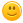# Confusion in Left CTL*

Is it right to assume that left CTL* is the same as CTL*?

No, it is not. The syntax is much richer, and there are many LeftCTL* formulas like E(Fa & Fb) which are not CTL formulas. However, any CTL formula belongs to LeftCTL*, so that CTL ice a strict (and tiny) subset of LeftCTL*. That much about syntax.

When it comes to semantics, then one can prove that for every LeftCTL* formula, there is an equivalent CTL formula. So, in terms of expressiveness both are the same.

Therefore, be careful what you mean with "the same".by (162k points)
I am not talking about CTL but CTL*.
Then, LeftCTL* is a strict subset of CTL* (both from the viewpoint of syntax and semantics, since its expressiveness is that of CTL which cannot express EGFa, for instance).